#### [区块链] 密码学——椭圆曲线密码算法（ECC）

1. 数学上的椭圆曲线及相关概念

2. 密码学中的椭圆曲线

3. 椭圆曲线上的加密/解密

4. 椭圆曲线签名与验证签名

1.1  从平行线谈起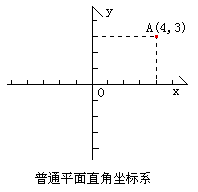▲直线L上的无穷远点只能有一个。（从定义可直接得出）
▲平面上一组相互平行的直线有公共的无穷远点。（从定义可直接得出）
▲ 平面上任何相交的两直线L1,L2有不同的无穷远点。（否则L1和L2有公共的无穷远点P ，则L1和L2有两个交点A、P，故假设错误。）
▲平面上全体无穷远点构成一条无穷远直线。（自己想象一下这条直线吧）
▲平面上全体无穷远点与全体平常点构成射影平面。

1.2  射影平面坐标系

（为什么？提示：可以从斜率考虑，因为平行线斜率相同）；

1.3  椭圆曲线

▲[1-1] 是Weierstrass方程（维尔斯特拉斯，Karl Theodor Wilhelm Weierstrass,1815-1897），是一个齐次方程。

▲ 椭圆曲线的形状，并不是椭圆的。只是因为椭圆曲线的描述方程，类似于计算一个椭圆周长的方程，故得名。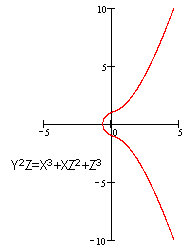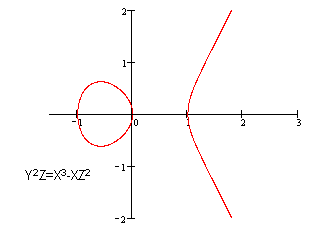▲ 所谓“非奇异”或“光滑”的，在数学中是指曲线上任意一点的偏导数Fx(x,y,z)，Fy(x,y,z)，Fz(x,y,z)不能同时为0。如果你没有学过高等数学，可以这样理解这个词，即满足方程的任意一点都存在切线。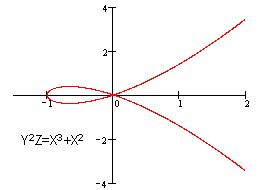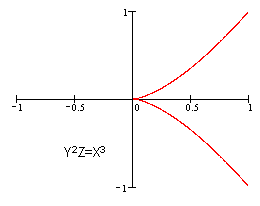▲椭圆曲线上有一个无穷远点O∞（0:1:0），因为这个点满足方程[1-1]。

y2+a1xy+a3y = x3+a2x2+a4x+a6 -------------------------[1-2]

Fx(x,y)= a1y-3x2-2a2x-a4
Fy(x,y)= 2y+a1x +a3

= (3x2+2a2x+a4-a1y) /(2y+a1x +a3)

1.4  椭圆曲线上的加法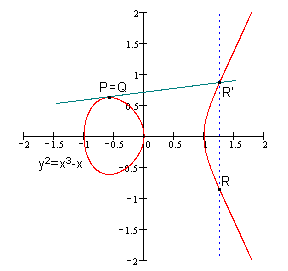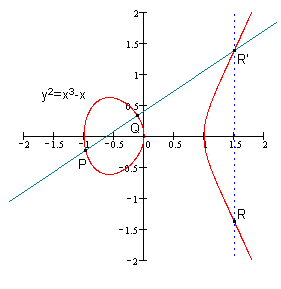▲这里的+不是实数中普通的加法，而是从普通加法中抽象出来的加法，他具备普通加法的一些性质，但具体的运算法则显然与普通加法不同。

▲根据这个法则，可以知道椭圆曲线无穷远点O∞与椭圆曲线上一点P的连线交于P’，过P’作y轴的平行线交于P，所以有 无穷远点 O∞+ P = P 。这样，无穷远点 O∞的作用与普通加法中零的作用相当（0+2=2），我们把无穷远点 O∞ 称为 零元。同时我们把P’称为P的负元（简称，负P；记作，-P）。（参见下图）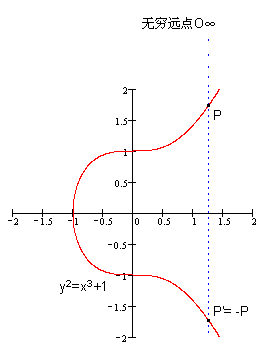▲根据这个法则，可以得到如下结论 ：如果椭圆曲线上的三个点A、B、C，处于同一条直线上，那么他们的和等于零元，即A+B+C= O∞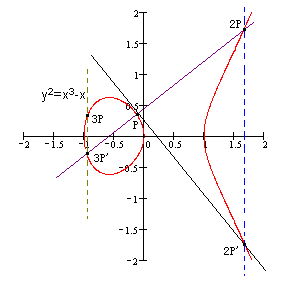▲k个相同的点P相加，我们记作kP。如下图：P+P+P = 2P+P = 3P。k=(3x2+2a2x+a4 -a1y) /(2y+a1x+a3)

y2+a1xy+a3y=x3+a2x2+a4x+a6    -----------------
y=(kx+b)                     -----------------

(kx+b)2+a1x(kx+b)+a3(kx+b) =x3+a2x2+a4x+a6    --------

x3=k2+ka1+a2+x1+x2;---------------------求出点-R的横坐标

y3=y1-k(x1-x3);-------------------------------求出点-R的纵坐标

（2）利用-R求R

-(a1x+a3)=y3+y4

x4=k2+ka1+a2+x1+x2;
y4=k(x1-x4)-y1-a1x4-a3;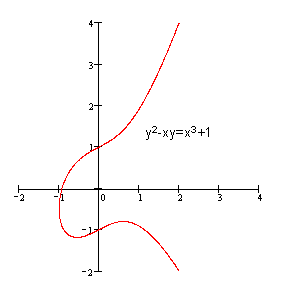Fp中只有p（p为素数）个元素0,1,2 …… p-2,p-1；
Fp 的加法（a+b）法则是 a+b≡c (mod p)；即，(a+b)÷p的余数 和c÷p的余数相同。
Fp 的乘法(a×b)法则是  a×b≡c (mod p)；
Fp 的除法(a÷b)法则是  a/b≡c (mod p)；即 a×b-1≡c  (mod p)；（b-1也是一个0到p-1之间的整数，但满足b×b-1≡1 (mod p) ）。
Fp 的单位元是1，零元是 0。

4a3+27b2≠0　(mod p)

y2=x3+ax+b  (mod p)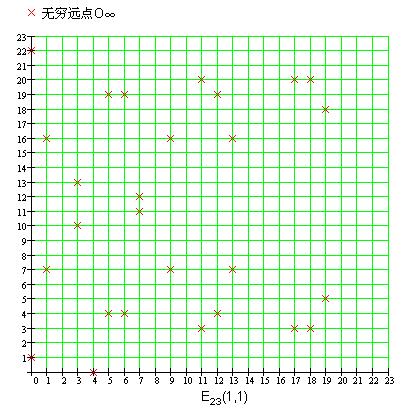Fp上的椭圆曲线同样有加法，但已经不能给以几何意义的解释。不过，加法法则和实数域上的差不多，请读者自行对比。

1. 无穷远点 O∞是零元，有O∞+ O∞= O∞，O∞+P=P
2. P(x,y)的负元是 (x,-y)，有P+(-P)= O∞
3. P(x1,y1),Q(x2,y2)的和R(x3,y3) 有如下关系：
x3≡k2-x1-x2(mod p)
y3≡k(x1-x3)-y1(mod p)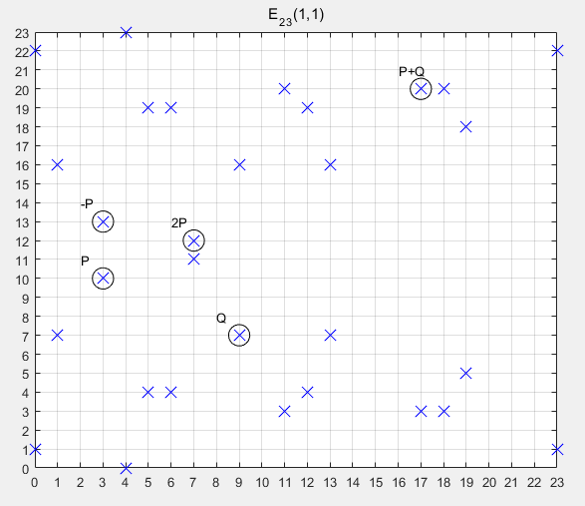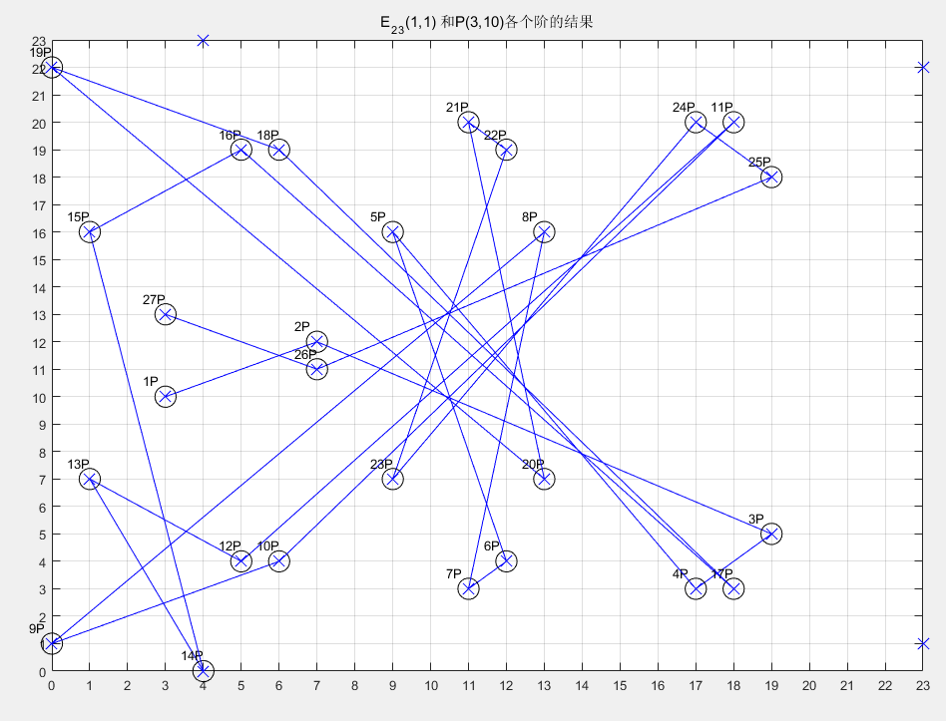K=kG  [其中 K,G为Ep(a,b)上的点，k为小于n（n是点G的阶）的整数]

k（k<n，n为基点g的阶）称为私有密钥（privte key），

k称为公开密钥（public="" key)。<="" p="">

1、用户A选定一条椭圆曲线Ep(a,b)，并取椭圆曲线上一点，作为基点G。
2、用户A选择一个私有密钥k，并生成公开密钥K=kG。
3、用户A将Ep(a,b)和点K，G传给用户B。
4、用户B接到信息后 ，将待传输的明文编码到Ep(a,b)上一点M（编码方法很多，这里不作讨论），并产生一个随机整数r（r<n）。
5、用户B计算点C1=M+rK；C2=rG。
6、用户B将C1、C2传给用户A。
7、用户A接到信息后，计算C1-kC2，结果就是点M。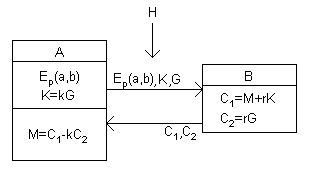总结：  设私钥、公钥分别为k、K，即K = kG，其中G为G点。

公钥加密：
选择随机数r，将消息M生成密文C，该密文是一个点对，即：
C = {rG, M+rK}，其中K为公钥

私钥解密：
M + rK - k(rG) = M + r(kG) - k(rG) = M
其中k、K分别为私钥、公钥。

ECC技术要求：

T=(p,a,b,G,n,h)。
（p 、a 、b 用来确定一条椭圆曲线，G为基点，n为点G的阶，h 是椭圆曲线上所有点的个数m与n相除的整数部分）

1、p 当然越大越安全，但越大，计算速度会变慢，200位左右可以满足一般安全要求；
2、p≠n×h；
3、pt≠1 (mod n)，1≤t<20；
4、4a3+27b2≠0 (mod p)；
5、n 为素数；
6、h≤4。

椭圆曲线签名算法，即ECDSA。

1、选择随机数r，计算点rG(x, y)。
2、根据随机数r、消息M的哈希h、私钥k，计算s = (h + kx)/r。
3、将消息M、和签名{rG, s}发给接收方。

1、接收方收到消息M、以及签名{rG=(x,y), s}。
2、根据消息求哈希h。
3、使用发送方公钥K计算：hG/s + xK/s，并与rG比较，如相等即验签成功。

hG/s + xK/s = hG/s + x(kG)/s = (h+xk)G/s
= r(h+xk)G / (h+kx) = rG

1、选择一条椭圆曲线Ep(a,b)，和基点G；
2、选择私有密钥k（k　　3、产生一个随机整数r（r　　4、将用户名和点R的坐标值x,y作为参数，计算SHA（Secure Hash Algorithm 安全散列算法，类似于MD5）值，即Hash=SHA(username,x,y)；
5、计算sn≡r - Hash * k (mod n)

1、从用户输入的序列号中，提取sn以及Hash；
2、计算点R≡sn*G+Hash*K ( mod p )，如果sn、Hash正确，其值等于软件作者签名过程中点R(x,y)的坐标，因为
sn≡r-Hash*k （mod n）

sn*G + Hash*K
=(r-Hash*k)*G+Hash*K
=rG-Hash*kG+Hash*K
=rG- Hash*K+ Hash*K
=rG=R ；
4、如果H=Hash 则注册成功。如果H≠Hash ，则注册失败(为什么？提示注意点R与Hash的关联性)。

Cracker要想制作注册机，只能通过软件中的Ep(a,b)，点G，公开密钥K ，并利用K=kG这个关系获得k后，才可以。而求k是很困难的。

1、选择一条椭圆曲线Ep(a,b)，和基点G；
5、计算 x’=x  (mod n)
6、计算sn≡(Hash+x’*k)/r (mod n)

1、从用户输入的序列号中，提取sn以及x’；
3、计算 R=(Hash*G+x’*K)/sn，如果sn、Hash正确,其值等于软件作者签名过程中点R(x,y)，因为
sn≡(Hash+x’*k)/r (mod n)

(Hash*G+x’*K)/sn
=(Hash*G+x’*K)/[(Hash+x’*k)/r]
=(Hash*G+x’*K)/[(Hash*G+x’*k*G)/(rG)]
=rG*[(Hash*G+x’*K)/(Hash*G+x’*K)]
=rG=R (mod p)
4、v≡x (mod n)
5、如果v=x’ 则注册成功。如果v≠x’ ，则注册失败。